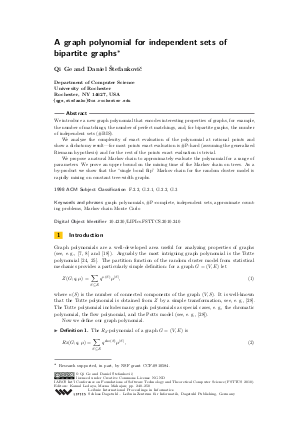Document# A graph polynomial for independent sets of bipartite graphs

### Authors Qi Ge, Daniel Stefankovic## File

LIPIcs.FSTTCS.2010.240.pdf
• Filesize: 482 kB
• 11 pages

## Cite As

Qi Ge and Daniel Stefankovic. A graph polynomial for independent sets of bipartite graphs. In IARCS Annual Conference on Foundations of Software Technology and Theoretical Computer Science (FSTTCS 2010). Leibniz International Proceedings in Informatics (LIPIcs), Volume 8, pp. 240-250, Schloss Dagstuhl - Leibniz-Zentrum für Informatik (2010)
https://doi.org/10.4230/LIPIcs.FSTTCS.2010.240

## Abstract

We introduce a new graph polynomial that encodes interesting properties of graphs, for example, the number of matchings, the number of perfect matchings, and, for bipartite graphs, the number of independent sets (#BIS). We analyze the complexity of exact evaluation of the polynomial at rational points and show a dichotomy result---for most points exact evaluation is #P-hard (assuming the generalized Riemann hypothesis) and for the rest of the points exact evaluation is trivial. We propose a natural Markov chain to approximately evaluate the polynomial for a range of parameters. We prove an upper bound on the mixing time of the Markov chain on trees. As a by-product we show that the ``single bond flip'' Markov chain for the random cluster model is rapidly mixing on constant tree-width graphs.
##### Keywords
• graph polynomials
• #P-complete
• independent sets
• approximate counting problems
• Markov chain Monte Carlo

## Metrics

• Access Statistics
• Total Accesses (updated on a weekly basis)
0